## August 28, 2011

### What if PageRank didn’t exist?

Filed under: data analysis,education,mathematics — Peter Saveliev @ 10:30 am

Gotcha! You thought this is about SEO. No, I meant “exist” in the mathematical sense…

When an entity or a concept in mathematics is introduced via a description instead of a direct computation, there is a possibility that this entity doesn’t even exist. This happens when there is nothing that satisfies the description. For example, “define x as a number that satisfies equation x+1=x” produces nothing.

Similarly, when something is introduced via an iterative process instead of a direct computation, does it always make sense? It’s possible that no matter how many iterations you run, you don’t get anything in particular (“divergence”). For example, “start with 1, then add 1/2, then add 1/3, 1/4, etc” leads to unlimited growth with no end.

So, what does this have to do with PageRank?

Let’s review the math behind it. Suppose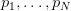$p_1,\ldots ,p_N$ are the$N$ pages/nodes in the graph (of the web). Suppose also that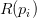$R(p_i)$ is the PageRank of node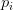$p_i$ and together they form a vector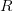$R$. Then the ranking satisfy the “balance equation”:$R(p_i) = d \sum_{p_j \in S(p_i)} \frac{R (p_j)}{L(p_j)} + \frac{1-d}{N},$
where
*$S(p_i)$ is the set of nodes that (inbound) link to$p_i$,
*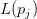$L(p_j)$ is the number of (outbound) links from node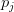$p_j$.

Skipping some algebra here, we discover that the PageRank vector$R$ is found as:$R = d(1-d) \left( \frac{1}{d}I-M \right) ^{-1}R_0,$
where the matrix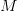$M$ is$M_{ij} = \begin{cases} 1 /L(p_j) & \mbox{if }p_j \in S(p_i),\ \\ 0 & \mbox{if }p_j \notin S(p_i), \end{cases}$
and$R_0(p_i) = \frac{1}{N},i=1,2,\ldots ,N,$ is the initial distribution of rankings.

Problem solved! But before we start celebrating we have to make sure that the inverse matrix in the formula exists!

The Perron–Frobenius theorem ensures that this is the case — but the matrix has to be “irreducible”.

Matrix$M$ is irreducible if and only if the graph with an edge from node$i$ to node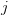$j$ when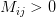$M_{ij} > 0$ is ”strongly connected”, i.e., there is a path from each node to every other node.

But we all know that there are always pages with no inbound links! The proof fails.

So, we have no reason to believe that a PageRank distribution exists for the given state of the web. This means that, no matter how you compute$R$, what comes out will be garbage.

Well, some people aren’t worried about this. Others worry so much that they try to fix it, in ridiculous ways. A common approach, for example, is to add “missing” links. Don’t get me started on that. If you introduce made-up parameters or try to make data fit your model, that’s bad math!

Are there other mathematical problems with PageRank? Still to come.

## August 23, 2011

### Modeling with discrete exterior calculus

Summer is over and I would like to report on a student’s research project that I especially liked. The full title is of the project was

Modeling Heat Transfer on Various Grids with Discrete Exterior Calculus by Julie Lang.

The main issue we ended up studying was isotropy as heat is supposed to transfer equally in all directions. Since no grid is isotropic, this is a big issue. Every time, we would start with all the initial heat concentrated in a single cell and then saw if, after many iterations, the shape of the heat function is circular. If it wasn’t, the model was simply wrong! Every time we had to come up with a modification and then continue with trial and error. Not very rigorous but fascinating scientifically. The last grid was rhomboidal and we were left with an oval, unfortunately…

It’s a good story and an interesting read. This is the link to the project page.

## August 2, 2011

### The math of PageRank: more bad news

Filed under: data analysis,education,mathematics,rants,reviews — Peter Saveliev @ 11:55 pm

Every time I get to read more about PageRank I run in serious issues with its math (previous posts here and here).

Briefly, the probabilistic interpretation of PageRank is invalid.

According to Brin/Page paper from 1998, “The probability that the random surfer visits a page is its PageRank.” And “We assume page A has pages T1…Tn which point to it (i.e., are citations). The parameter d is a damping factor which can be set between 0 and 1… Also C(A) is defined as the number of links going out of page A. The PageRank of a page A is given as follows:

 PR(A) = (1-d) + d (PR(T1)/C(T1) + ... + PR(Tn)/C(Tn))

Note that the PageRanks form a probability distribution over web pages, so the sum of all web pages’ PageRanks will be one.”

When I decided to implement the PageRank algorithm a few days ago, I quickly discovered that it should be (1-d)/n instead of (1-d). That’s a typo, fine. But, after fixing this, I wasted half a day trying to figure out why the ranks still wouldn’t add up to 1. Turns out, it was all a lie: they don’t have to!

I don’t understand now how I missed that. If there is only one page, its PageRank has to be 1-d. If there are two pages with T linked to A and nothing else, then we can assign: T=0 and A=(1-d)/2. They don’t have to add up to 1!

Best one can say is this. If one uses the above formula for iteration and starts with a distribution, then yes, you will get a distribution after every iteration… But only if there are no link-less pages!

To get out of this trouble, there have been some tweaks suggested. Hard to tell which one is sillier:

• normalizing (dividing by the sum of all PageRanks) and
• assuming that no links means that the page links to every page.

That’s what happens when you try to make data fit the model.

After more than 10 years of usage, PageRank’s paradigm is clear. The people who care about their PageRank – millions of web site owners – understand what it is about. It isn’t about probability. PageRank is about passing something valuable from a page to the next along its links. This something has been called popularity, significance, authority, “link juice”, etc.

So, PageRank shows who, after all this “redistribution of wealth”, ends up on top. This seems to make quite a lot of sense, doesn’t it?

Unfortunately, the actual computations lead to problems.

In the presence of cycles of links, the algorithm diverges to infinity. This lead to the introduction of the damping factor (bad math), which didn’t prevent the gaming of the algorithm. Google’s answer was surreptitious tweaking, attempts to evaluate the “quality” of sites, manual interventions, on and on.

Even though this problem certainly isn’t a new discovery, the myth clearly lives on: Wikipedia says “PageRank is a probability distribution used to represent the likelihood that a person randomly clicking on links will arrive at any particular page.” It then proceeds to give an example in the next section that isn’t a distribution.

I’ve learned my lesson: bad math simply doesn’t deserve mathematical analysis. Once it’s determined that the idea is bad math, you know it’s a dead end! Instead, try to find an alternative. The acyclic rank still to come…